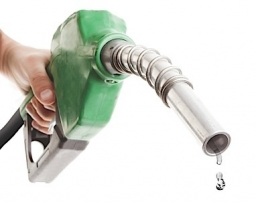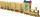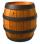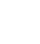# Gasoline-oil ratio

The manufacturer of a scooter engine recommends a gasoline-oil fuel mixture ratio of 15 to 1. In a particular garage, we can buy pure gasoline and a gasoline-oil mixture, which is 75% gasoline.

How much gasoline and how much of the gasoline-oil mix do we need to make 8.0 L of fuel for the scooter engine?

Result

a =  6 l
b =  2 l

#### Solution:

a+b = 8.0
(1-0.75)*b = 8.0 * (1/(1+15))

a+b = 8
0.25b = 0.5

a = 6
b = 2

Calculated by our linear equations calculator.
$b = 2 = 2 \ \text { l }$Our examples were largely sent or created by pupils and students themselves. Therefore, we would be pleased if you could send us any errors you found, spelling mistakes, or rephasing the example. Thank you!

Leave us a comment of this math problem and its solution (i.e. if it is still somewhat unclear...):Be the first to comment!#### Following knowledge from mathematics are needed to solve this word math problem:

Need help calculate sum, simplify or multiply fractions? Try our fraction calculator. Our percentage calculator will help you quickly calculate various typical tasks with percentages. Do you have a linear equation or system of equations and looking for its solution? Or do you have quadratic equation? Tip: Our volume units converter will help you with the conversion of volume units.

## Next similar math problems:

1. Gasoline canisters35 liters of gasoline is to be divided into 4 canisters so that in the third canister will have 5 liters less than in the first canister, the fourth canister 10 liters more than the third canister and the second canister half of that in the first canist
2. Boys to girlsThe ratio of boys to girls in a party is 3:5 . If 6 more boys arrived and 4 girls left the party, the ratio of boys to girls would be 5:6 . How many are in the party originally?
3. SchoolSchool attend 792 children, boys and girls ratio is 4:5. How many more girls go to school (than boys)?
4. Percentage 1052 shorts and missed 13. Calculate percentage
5. Flowers 2Cha cruz has a garden. The ratio roses to tulips is 2 : 5, the ratio of roses to orchids is 7 : 6. Cha cruz wonders what the ratio of tulips to orchids is. If Cha cruz has 183 plants, how many of each kind are there?
6. Linsys2Solve two equations with two unknowns: 400x+120y=147.2 350x+200y=144
7. Ball gameRichard, Denis and Denise together scored 932 goals. Denis scored 4 goals over Denise but Denis scored 24 goals less than Richard. Determine the number of goals for each player.
8. EmployeesThere are 1116 people working in three factory halls. In the first one, there are 18% more than the third, and 60 persons more than the second. How many employees work in individual halls?
9. ChildrenThe group has 42 children. There are 4 more boys than girls. How many boys and girls are in the group?
10. Three workshopsThere are 2743 people working in three workshops. In the second workshop works 140 people more than in the first and in third works 4.2 times more than the second one. How many people work in each workshop?55%+36%+88%+71%+100=63% what is whole (X)? Percents can be added directly together if they are taken from the same whole, which means they have the same base amount. .. . You would add the two percentages to find the total amount.The temporary workers planted new trees. Of the total number of 500 seedlings, they managed to plant 426. How many percents did they meet the daily planting limit?We want to prove the sentence: If the natural number n is divisible by six, then n is divisible by three. From what assumption we started?There are 370 employees in the company - women are 15% less than men. How many men work in the company?The barrel is filled with water up to 57% of its capacity. When we add two buckets will be filled to 73% of its capacity. What is the volume of a barrel, if the volume of the bucket is 12 liters?Solve the system of linear equations with three unknowns: A + B + C = 14 B - A - C = 4 2A - B + C = 0Simiyu and Nasike each collected a number of stones in an arithmetic lesson. If Simiyu gave Nasike 5 stones, Nasike would have twice as many stones as Simiyu. If initially, Simiyu had five stones less than Nasike how many stones did each have?